# Fit Custom Distributions

This example shows how to fit a custom distribution to univariate data by using the `mle` function.

You can use the `mle` function to compute maximum likelihood parameter estimates and to estimate their precision for built-in distributions and custom distributions. To fit a custom distribution, you need to define a function for the custom distribution in a file or by using an anonymous function. In the simplest cases, you can write code to compute the probability density function (pdf) or logarithm of pdf for the distribution that you want to fit, and then call `mle` to fit the distribution. This example covers the following cases using the pdf or logarithm of pdf:

• Fitting a distribution for truncated data

• Fitting a mixture of two distributions

• Fitting a weighted distribution

• Finding accurate confidence intervals of parameter estimates for small-sized samples using parameter transformation

Note that you can use the TruncationBounds name-value argument of `mle` for truncated data instead of defining a custom function. Also, for a mixture of two normal distributions, you can use the `fitgmdist` function. This example uses the `mle` function and a custom function for these cases.

### Fit Zero-Truncated Poisson Distribution

Count data is often modeled using a Poisson distribution, and you can use the `poissfit` or `fitdist` function to fit a Poisson distribution. However, in some situations, counts that are zero are not recorded in the data, so fitting a Poisson distribution is not straightforward because of the missing zeros. In this case, fit a Poisson distribution to zero-truncated data by using the `mle` function and a custom distribution function.

First, generate some random Poisson data.

```rng(18,'twister') % For reproducibility lambda = 1.75; n = 75; x1 = poissrnd(lambda,n,1);```

Next, remove all the zeros from the data to simulate the truncation.

`x1 = x1(x1 > 0);`

Check the number of samples in `x1` after truncation.

`length(x1)`
```ans = 65 ```

Plot a histogram of the simulated data.

`histogram(x1,0:1:max(x1)+1)`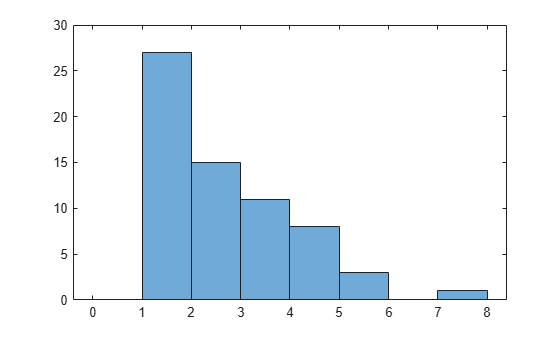The data looks like a Poisson distribution except it contains no zeros. You can use a custom distribution that is identical to a Poisson distribution on the positive integers, but has no probability at zero. By using a custom distribution, you can estimate the Poisson parameter `lambda` while accounting for the missing zeros.

You need to define the zero-truncated Poisson distribution by its probability mass function (pmf). Create an anonymous function to compute the probability for each point in `x1`, given a value for the Poisson distribution's mean parameter `lambda`. The pmf for a zero-truncated Poisson distribution is the Poisson pmf normalized so that it sums to one. With zero truncation, the normalization is `1–Probability(x1<0)`.

`pf_truncpoiss = @(x1,lambda) poisspdf(x1,lambda)./(1-poisscdf(0,lambda));`

For simplicity, assume that all the `x1` values given to this function are positive integers, with no checks. For error checking or a more complicated distribution that takes more than a single line of code, you must define the function in a separate file.

Find a reasonable rough first guess for the parameter `lambda`. In this case, use the sample mean.

`start = mean(x1)`
```start = 2.2154 ```

Provide `mle` with the data, custom pmf function, initial parameter value, and lower bound of the parameter. Because the mean parameter of the Poisson distribution must be positive, you also need to specify a lower bound for `lambda`. The `mle` function returns the maximum likelihood estimate of `lambda`, and optionally, the approximate 95% confidence intervals for the parameters.

```[lambdaHat,lambdaCI] = mle(x1,'pdf',pf_truncpoiss,'Start',start, ... 'LowerBound',0)```
```lambdaHat = 1.8760 ```
```lambdaCI = 2×1 1.4990 2.2530 ```

The parameter estimate is smaller than the sample mean. The maximum likelihood estimate accounts for the zeros not present in the data.

Alternatively, you can specify the truncation bounds by using the TruncationBounds name-value argument.

```[lambdaHat2,lambdaCI2] = mle(x1,'Distribution','Poisson', ... 'TruncationBounds',[0 Inf])```
```lambdaHat2 = 1.8760 ```
```lambdaCI2 = 2×1 1.4990 2.2530 ```

You can also compute a standard error estimate for `lambda` by using the large-sample variance approximation returned by `mlecov`.

```avar = mlecov(lambdaHat,x1,'pdf',pf_truncpoiss); stderr = sqrt(avar)```
```stderr = 0.1923 ```

To visual check the fit, plot the fitted pmf against a normalized histogram of the raw data

```histogram(x1,'Normalization','pdf') xgrid = min(x1):max(x1); pmfgrid = pf_truncpoiss(xgrid,lambdaHat); hold on plot(xgrid,pmfgrid,'-') xlabel('x1') ylabel('Probability') legend('Sample Data','Fitted pmf','Location','best') hold off```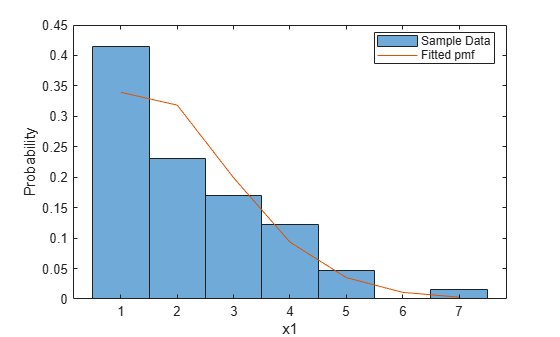### Fit Upper-Truncated Normal Distribution

Continuous data can sometimes be truncated. For example, observations larger than some fixed value might not be recorded because of limitations in data collection.

In this case, simulate data from a truncated normal distribution. First, generate some random normal data.

```n = 500; mu = 1; sigma = 3; rng('default') % For reproducibility x2 = normrnd(mu,sigma,n,1);```

Next, remove any observations that fall beyond the truncation point `xTrunc`. Assume that `xTrunc` is a known value that you do not need to estimate.

```xTrunc = 4; x2 = x2(x2 < xTrunc);```

Check the number of samples in `x2` after truncation.

`length(x2)`
```ans = 430 ```

Create a histogram of the simulated data.

`histogram(x2)`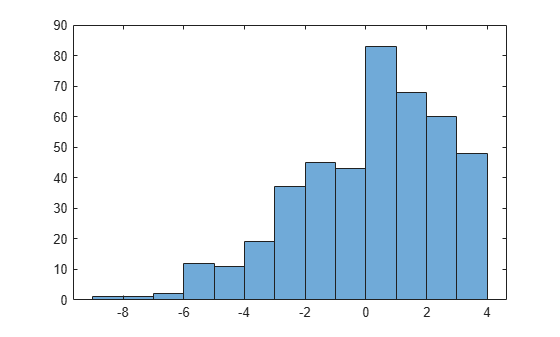Fit the simulated data with a custom distribution that is identical to a normal distribution for `x2 < xTrunc`, but has zero probability above `xTrunc`. By using a custom distribution, you can estimate the normal parameters `mu` and `sigma` while accounting for the missing tail.

Define the truncated normal distribution by its pdf. Create an anonymous function to compute the probability density value for each point in x, given values for the parameters `mu` and `sigma`. With the truncation point fixed and known, the pdf for a truncated normal distribution is the pdf truncated and then normalized so that it integrates to one. The normalization is the cdf evaluated at `xTrunc`. For simplicity, assume that all `x2` values are less than `xTrunc`, without checking.

```pdf_truncnorm = @(x2,mu,sigma) ... normpdf(x2,mu,sigma)./normcdf(xTrunc,mu,sigma);```

Because you do not need to estimate the truncation point `xTrunc`, it is not included with the input distribution parameters of the custom pdf function. `xTrunc` is also not part of the data vector input argument. An anonymous function can access variables in the workspace, so you do not have to pass `xTrunc` to the anonymous function as an additional argument.

Provide a rough starting guess for the parameter estimates. In this case, because the truncation is not extreme, use the sample mean and standard deviation.

`start = [mean(x2),std(x2)]`
```start = 1×2 0.1585 2.4125 ```

Provide `mle` with the data, custom pdf function, initial parameter values, and lower bounds of the parameters. Because `sigma` must be positive, you also need to specify lower parameter bounds. `mle` returns the maximum likelihood estimates of `mu` and `sigma` as a single vector, as well as a matrix of approximate 95% confidence intervals for the two parameters.

```[paramEsts,paramCIs] = mle(x2,'pdf',pdf_truncnorm,'Start',start, ... 'LowerBound',[-Inf 0])```
```paramEsts = 1×2 1.1298 3.0884 ```
```paramCIs = 2×2 0.5713 2.7160 1.6882 3.4607 ```

The estimates of `mu` and `sigma` are larger than the sample mean and standard deviation. The model fit accounts for the missing upper tail of the distribution.

Alternatively, you can specify the truncation bounds by using the TruncationBounds name-value argument.

```[paramEsts2,paramCIs2] = mle(x2,'Distribution','Normal', ... 'TruncationBounds',[-Inf xTrunc])```
```paramEsts2 = 1×2 1.1297 3.0884 ```
```paramCIs2 = 2×2 0.5713 2.7160 1.6882 3.4607 ```

You can compute an approximate covariance matrix for the parameter estimates using `mlecov`. The approximation typically works well for large samples, and you can approximate the standard errors by the square roots of the diagonal elements.

`acov = mlecov(paramEsts,x2,'pdf',pdf_truncnorm)`
```acov = 2×2 0.0812 0.0402 0.0402 0.0361 ```
`stderr = sqrt(diag(acov))`
```stderr = 2×1 0.2849 0.1900 ```

To visually check the fit, plot the fitted pdf against a normalized histogram of the raw data.

```histogram(x2,'Normalization','pdf') xgrid = linspace(min(x2),max(x2)); pdfgrid = pdf_truncnorm(xgrid,paramEsts(1),paramEsts(2)); hold on plot(xgrid,pdfgrid,'-') xlabel('x2') ylabel('Probability Density') legend('Sample Data','Fitted pdf','Location','best') hold off```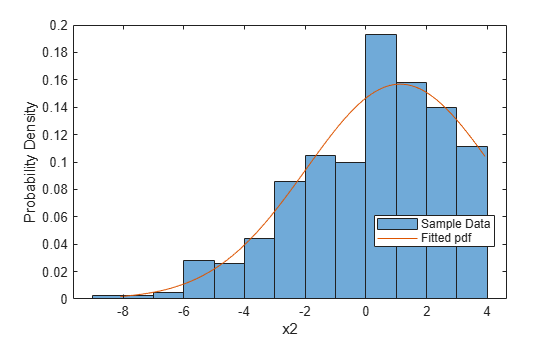### Fit Mixture of Two Normal Distributions

Some data sets exhibit bimodality, or even multimodality, and fitting a standard distribution to such data is usually not appropriate. However, a mixture of simple unimodal distributions can often model such data very well.

In this case, fit a mixture of two normal distributions to simulated data. Consider simulated data with the following constructive definition:

• First, flip a biased coin.

• If the coin lands on heads, pick a value at random from a normal distribution with mean ${\mu }_{1}$ and standard deviation ${\sigma }_{1}$.

• If the coin lands on tails, pick a value at random from a normal distribution with mean ${\mu }_{2}$ and standard deviation ${\sigma }_{2}$.

Generate a data set from a mixture of Student's t distributions instead of using the same model that you are fitting. By using different distributions, similar to a technique used in a Monte-Carlo simulation, you can test how robust a fitting method is to departures from the assumptions of the model being fit.

```rng(10) % For reproducibility x3 = [trnd(20,1,50) trnd(4,1,100)+3]; histogram(x3)```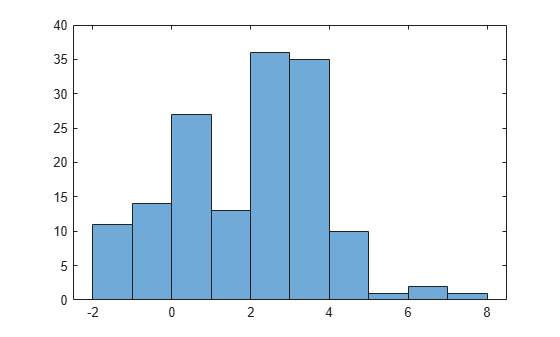Define the model to fit by creating an anonymous function that computes the probability density. The pdf for a mixture of two normal distributions is a weighted sum of the pdfs of the two normal components, weighted by the mixture probability. The anonymous function takes six inputs: a vector of data at which to evaluate the pdf and five distribution parameters. Each component has parameters for its mean and standard deviation.

```pdf_normmixture = @(x3,p,mu1,mu2,sigma1,sigma2) ... p*normpdf(x3,mu1,sigma1) + (1-p)*normpdf(x3,mu2,sigma2);```

You also need an initial guess for the parameters. Defining a starting point becomes more important as the number of model parameters increases. Here, start with an equal mixture (`p` = 0.5) of normal distributions, centered at the two quartiles of the data, with equal standard deviations. The starting value for the standard deviation comes from the formula for the variance of a mixture in terms of the mean and variance of each component.

```pStart = .5; muStart = quantile(x3,[.25 .75])```
```muStart = 1×2 0.3351 3.3046 ```
`sigmaStart = sqrt(var(x3) - .25*diff(muStart).^2)`
```sigmaStart = 1.1602 ```
`start = [pStart muStart sigmaStart sigmaStart];`

Specify bounds of zero and one for the mixing probability, and lower bounds of zero for the standard deviations. Set the remaining elements of the bounds vectors to `+Inf` or `–Inf`, to indicate no restrictions.

```lb = [0 -Inf -Inf 0 0]; ub = [1 Inf Inf Inf Inf]; paramEsts = mle(x3,'pdf',pdf_normmixture,'Start',start, ... 'LowerBound',lb,'UpperBound',ub)```
```Warning: Maximum likelihood estimation did not converge. Iteration limit exceeded. ```
```paramEsts = 1×5 0.3273 -0.2263 2.9914 0.9067 1.2059 ```

The warning message indicates that the function does not converge with the default iteration settings. Display the default options.

`statset('mlecustom')`
```ans = struct with fields: Display: 'off' MaxFunEvals: 400 MaxIter: 200 TolBnd: 1.0000e-06 TolFun: 1.0000e-06 TolTypeFun: [] TolX: 1.0000e-06 TolTypeX: [] GradObj: 'off' Jacobian: [] DerivStep: 6.0555e-06 FunValCheck: 'on' Robust: [] RobustWgtFun: [] WgtFun: [] Tune: [] UseParallel: [] UseSubstreams: [] Streams: {} OutputFcn: [] ```

The default maximum number of iterations for custom distributions is 200. Override the default to increase the number of iterations, using an options structure created with the `statset` function. Also, increase the maximum function evaluations.

```options = statset('MaxIter',300,'MaxFunEvals',600); paramEsts = mle(x3,'pdf',pdf_normmixture,'Start',start, ... 'LowerBound',lb,'UpperBound',ub,'Options',options)```
```paramEsts = 1×5 0.3273 -0.2263 2.9914 0.9067 1.2059 ```

The final iterations to convergence are significant only in the last few digits of the result. However, a best practice is to always make sure that convergence is reached.

To visually check the fit, plot the fitted density against a probability histogram of the raw data.

```histogram(x3,'Normalization','pdf') hold on xgrid = linspace(1.1*min(x3),1.1*max(x3),200); pdfgrid = pdf_normmixture(xgrid, ... paramEsts(1),paramEsts(2),paramEsts(3),paramEsts(4),paramEsts(5)); plot(xgrid,pdfgrid,'-') hold off xlabel('x3') ylabel('Probability Density') legend('Sample Data','Fitted pdf','Location','best')```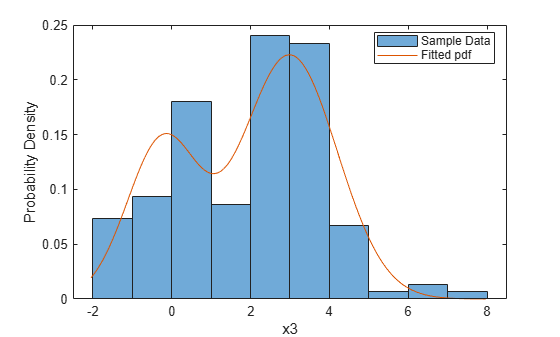Alternatively, for a mixture of normal distributions, you can use the `fitgmdist` function. The estimates can be different due to initial estimates and settings of the iterative algorithm.

`Mdl = fitgmdist(x3',2)`
```Mdl = Gaussian mixture distribution with 2 components in 1 dimensions Component 1: Mixing proportion: 0.329180 Mean: -0.2200 Component 2: Mixing proportion: 0.670820 Mean: 2.9975 ```
`Mdl.Sigma`
```ans = ans(:,:,1) = 0.8274 ans(:,:,2) = 1.4437 ```

### Fit Weighted Normal Distribution to Data with Unequal Precisions

Assume that you have 10 data points, where each point is actually the average of anywhere from one to eight observations. The original observations are not available, but the number of observations for each data point is known. The precision of each point depends on its corresponding number of observations. You need to estimate the mean and standard deviation of the raw data.

```x4 = [0.25 -1.24 1.38 1.39 -1.43 2.79 3.52 0.92 1.44 1.26]'; m = [8 2 1 3 8 4 2 5 2 4]';```

The variance of each data point is inversely proportional to its corresponding number of observations, so use `1/m` to weight the variance of each data point in a maximum likelihood fit.

`w = 1./m;`

In this model, you can define the distribution by its pdf. However, using a logarithm of pdf is more suitable, because the normal pdf has the form

``` c .* exp(-0.5 .* z.^2), ```

and `mle` takes the log of the pdf to compute the loglikelihood. So, instead, create a function that computes the logarithm of pdf directly.

The logarithm of pdf function must compute the logarithm of the probability density for each point in `x`, given normal distribution parameters `mu` and `sigma`. It also needs to account for the different variance weights. Define a function named `helper_logpdf_wn1` in a separate file `helper_logpdf_wn1.m`.

```function logy = helper_logpdf_wn1(x,m,mu,sigma) %HELPER_LOGPDF_WN1 Logarithm of pdf for a weight normal distribution % This function supports only the example Fit Custom Distributions % (customdist1demo.mlx) and might change in a future release. v = sigma.^2 ./ m; logy = -(x-mu).^2 ./ (2.*v) - .5.*log(2.*pi.*v); end ```

Provide a rough first guess for the parameter estimates. In this case, use the unweighted sample mean and standard deviation.

`start = [mean(x4),std(x4)]`
```start = 1×2 1.0280 1.5490 ```

Because `sigma` must be positive, you need to specify lower parameter bounds.

```[paramEsts1,paramCIs1] = mle(x4,'logpdf', ... @(x,mu,sigma)helper_logpdf_wn1(x,m,mu,sigma), ... 'Start',start,'LowerBound',[-Inf,0])```
```paramEsts1 = 1×2 0.6244 2.8823 ```
```paramCIs1 = 2×2 -0.2802 1.6191 1.5290 4.1456 ```

The estimate of `mu` is less than two-thirds of the estimate of the sample mean. The estimate is influenced by the most reliable data points, that is, the points based on the largest number of raw observations. In this data set, those points tend to pull the estimate down from the unweighted sample mean.

### Fit Normal Distribution Using Parameter Transformation

The `mle` function computes confidence intervals for the parameters using a large-sample normal approximation for the distribution of the estimators if an exact method is not available. For small sample sizes, you can improve the normal approximation by transforming one or more parameters. In this case, transform the scale parameter of a normal distribution to its logarithm.

First, define a new log pdf function named `helper_logpdf_wn2` that uses a transformed parameter for `sigma`.

```function logy = helper_logpdf_wn2(x,m,mu,logsigma) %HELPER_LOGPDF_WN2 Logarithm of pdf for a weight normal distribution with % log(sigma) parameterization % This function supports only the example Fit Custom Distributions % (customdist1demo.mlx) and might change in a future release. v = exp(logsigma).^2 ./ m; logy = -(x-mu).^2 ./ (2.*v) - .5.*log(2.*pi.*v); end ```

Use the same starting point transformed to the new parameterization for `sigma`, that is, the log of the sample standard deviation.

`start = [mean(x4),log(std(x4))]`
```start = 1×2 1.0280 0.4376 ```

Because `sigma` can be any positive value, `log(sigma)` is unbounded, and you do not need to specify lower or upper bounds.

```[paramEsts2,paramCIs2] = mle(x4,'logpdf', ... @(x,mu,sigma)helper_logpdf_wn2(x,m,mu,sigma), ... 'Start',start)```
```paramEsts2 = 1×2 0.6244 1.0586 ```
```paramCIs2 = 2×2 -0.2802 0.6203 1.5290 1.4969 ```

Because the parameterization uses `log(sigma)`, you have to transform back to the original scale to get an estimate and confidence interval for `sigma`.

`sigmaHat = exp(paramEsts2(2))`
```sigmaHat = 2.8823 ```
`sigmaCI = exp(paramCIs2(:,2))`
```sigmaCI = 2×1 1.8596 4.4677 ```

The estimates for both `mu` and `sigma` are the same as in the first fit, because maximum likelihood estimates are invariant to parameterization. The confidence interval for `sigma` is slightly different from `paramCIs1(:,2)`.